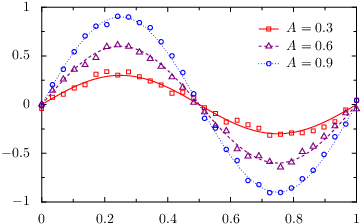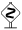# PyX — Example: graphs/join.py

0.6 KB
76.8 KB
1.1 KB
72.6 KB
38.5 KB
48.3 KB

## Joing multiple datasets for grouping in the graph key```from pyx import *

g = graph.graphxy(width=8, key=graph.key.key())

As = [0.3, 0.6, 0.9]

d = [graph.data.join([graph.data.function("y_a(x_a)=A*sin(2*pi*x_a)", context=dict(A=A)),
graph.data.file("join.dat", x_b=1, y_b=i+2)],
title=r"\$A=%g\$" % A)
for i, A in enumerate(As)]

g.plot(d,
[graph.style.pos(usenames=dict(x="x_a", y="y_a")),
graph.style.line(attrs),
graph.style.pos(usenames=dict(x="x_b", y="y_b")),
graph.style.symbol(graph.style.symbol.changesquare, symbolattrs=attrs, size=0.1)])

g.writeEPSfile()
g.writePDFfile()
g.writeSVGfile()
```

### Description

If you want to group datasets in the graph key, e.g., to compare simulation results with measurement data or some approximate results with results from a more detailed calculation, you can pass a list of datasets to the `graph.data.join` class.

When doing so, you have to assign unique names to the corresponding datasets. In the example, we have used `x_a` and `y_a` as well as `x_b` and `y_b` as names. Subsequently, one has to tell the graph style which data columns—identified by the given name—it has to plot. This can be done by inserting `graph.style.pos` instances before the respective graph style. By passing a dictionary to the `usenames` argument, one specifies the mapping from the data columns to the corresponding graph axes `x`, `y`, `x2`, and so on.When using the standard names `x`, `y`, etc. for the data columns, as is done in most cases, PyX does insert the `graph.style.pos` instance mapping those data columns to the axis with the same name, automatically.